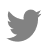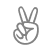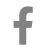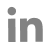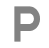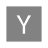# Data Analytics Glossary

Try: Cardinality(SQL), Raw Data, No SQL...

## Random Sample

#### What is a Random Sample?

A random sample is a kind of sampling technique that considers the same probability for each sample. It gives an unbiased representation of the total population. If it does not represent the population it is said to be a sampling error.

#### How does a Random Sample work?

Random sampling is a very simple form of gathering data out of a total population. Each member of a subset is given an equal chance of being selected in random sampling. For instance, the total staff in a company is 400, and to do a survey, 40 members would be selected to conduct the survey. These 40 members would be chosen randomly which most probably represent 100% of the staff. In case, it does not represent the whole population (staff) it would be called a sampling error.

#### Types and Examples:

The upper described scenario (400 members and 40 randomly selected) is an example of random data sampling.